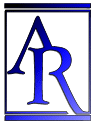Annu. Rev. Astron. Astrophys. 1984. 22: 157-184 Copyright © 1984 by Annual Reviews. All rights reserved

### 3. ALTERNATIVE VIEWS OF REDSHIFT

TIRED LIGHT     An alternative theory of light propagation is the "tired light" theory, in which light loses energy progressively while traveling across large distances of extragalactic space. Thus we abandon Equation 2; then the observed redshifts might occur in a static universe, where no SHBB occurs. When a detailed mechanism for such an effect is proposed, various problems occur (65); and attempts to check if it is true are negative (34).

ALTERNATIVE GEODESICS     Two-metric theories may involve light traveling on geodesics of another metric than that specifying length and time measurements; thus we effectively abandon Equation 2a. In general, these theories will still require different field equations or energy violation to avoid a singularity (see below). Furthermore, any such dynamic two-metric theory is likely to run into causality problems (77).

A particular case is Segal's theory (92), where in effect two metrics are laid down a priori from geometrical rather than physical principles (and are singularity free). The theory therefore proposes a cosmology independent of any gravitational equations; it demands a quadratic (magnitude, redshift) distance relation rather than the linear one predicted by the FLRW universes. The interpretation of the observations is the subject of a dispute centering on the nature of the selection effects and the statistics used (70, 93, 98); but the weight of the evidence seems to be against the quadratic effect. Additionally, the distribution of absorption lines in QSO spectra appears to rule these models out (81).